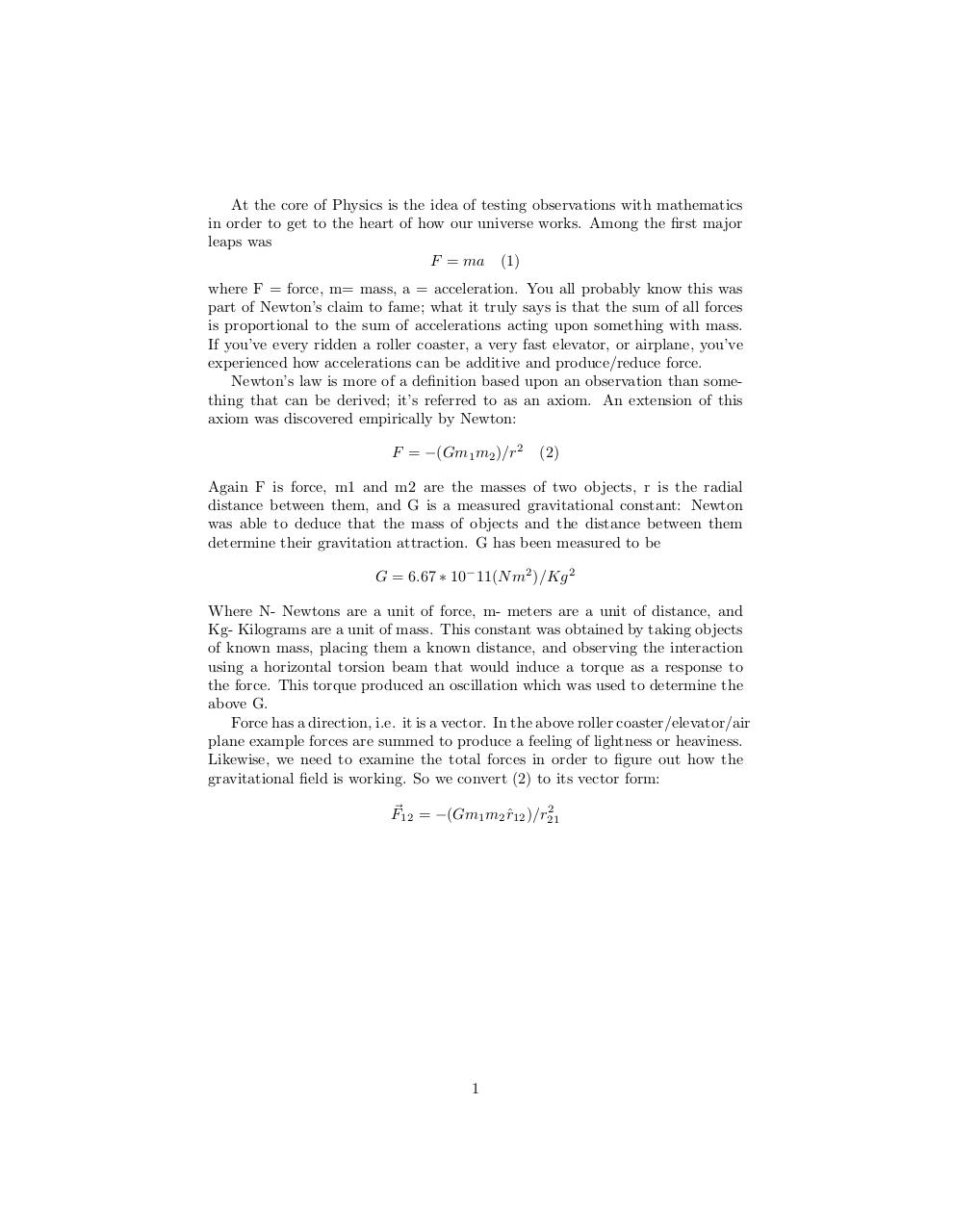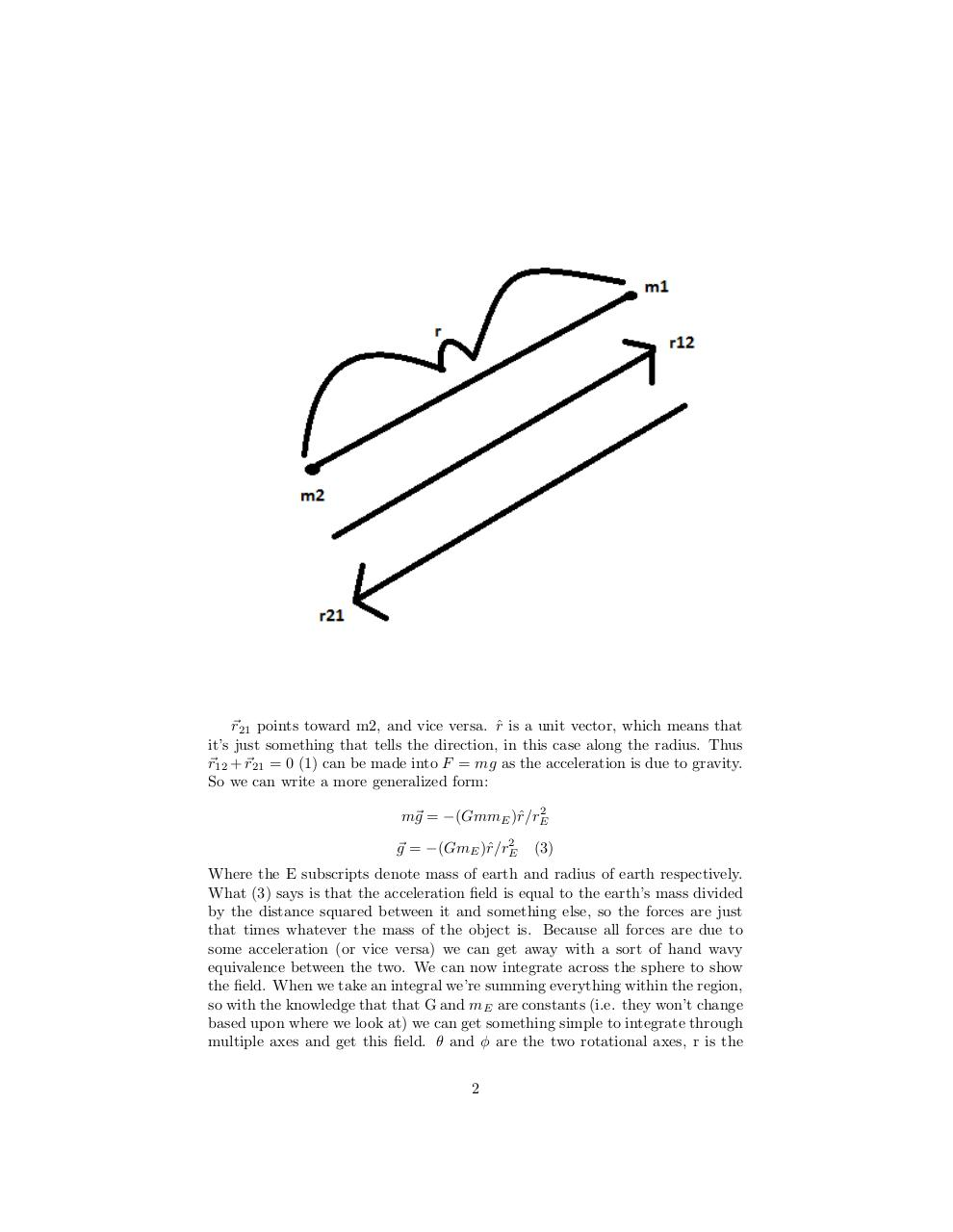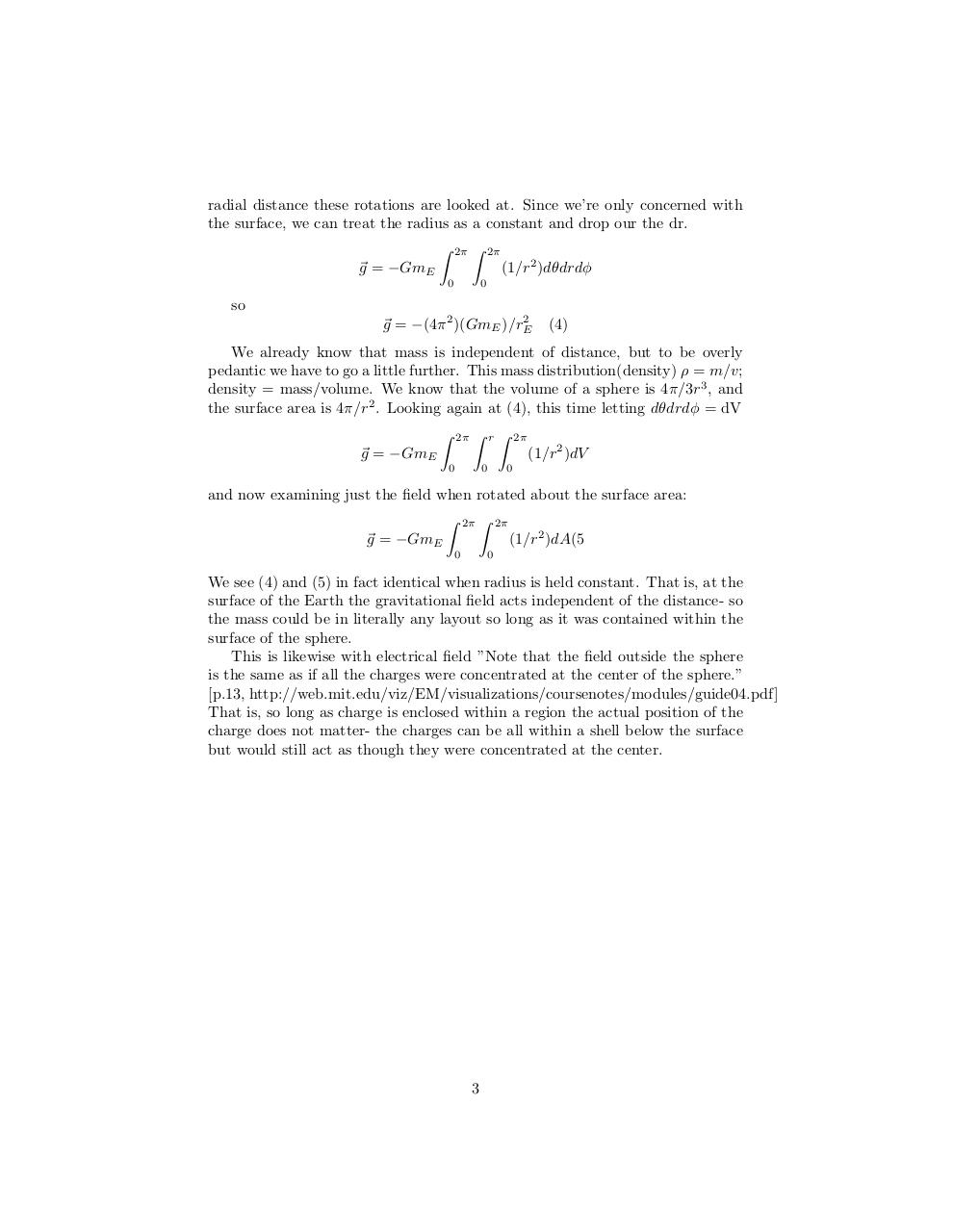# Fields .pdf

### File information

Original filename: Fields.pdf

This PDF 1.5 document has been generated by TeX / MiKTeX pdfTeX-1.40.15, and has been sent on pdf-archive.com on 09/09/2016 at 07:45, from IP address 107.146.x.x. The current document download page has been viewed 491 times.
File size: 82 KB (3 pages).
Privacy: public file

Fields.pdf (PDF, 82 KB)

### Document preview

At the core of Physics is the idea of testing observations with mathematics
in order to get to the heart of how our universe works. Among the first major
leaps was
F = ma (1)
where F = force, m= mass, a = acceleration. You all probably know this was
part of Newton’s claim to fame; what it truly says is that the sum of all forces
is proportional to the sum of accelerations acting upon something with mass.
If you’ve every ridden a roller coaster, a very fast elevator, or airplane, you’ve
experienced how accelerations can be additive and produce/reduce force.
Newton’s law is more of a definition based upon an observation than something that can be derived; it’s referred to as an axiom. An extension of this
axiom was discovered empirically by Newton:
F = −(Gm1 m2 )/r2

(2)

Again F is force, m1 and m2 are the masses of two objects, r is the radial
distance between them, and G is a measured gravitational constant: Newton
was able to deduce that the mass of objects and the distance between them
determine their gravitation attraction. G has been measured to be
G = 6.67 ∗ 10− 11(N m2 )/Kg 2
Where N- Newtons are a unit of force, m- meters are a unit of distance, and
Kg- Kilograms are a unit of mass. This constant was obtained by taking objects
of known mass, placing them a known distance, and observing the interaction
using a horizontal torsion beam that would induce a torque as a response to
the force. This torque produced an oscillation which was used to determine the
above G.
Force has a direction, i.e. it is a vector. In the above roller coaster/elevator/air
plane example forces are summed to produce a feeling of lightness or heaviness.
Likewise, we need to examine the total forces in order to figure out how the
gravitational field is working. So we convert (2) to its vector form:
2
F~12 = −(Gm1 m2 rˆ12 )/r21

1

~r21 points toward m2, and vice versa. rˆ is a unit vector, which means that
it’s just something that tells the direction, in this case along the radius. Thus
~r12 + ~r21 = 0 (1) can be made into F = mg as the acceleration is due to gravity.
So we can write a more generalized form:
2
m~g = −(GmmE )ˆ
r/rE
2
~g = −(GmE )ˆ
r/rE

(3)

Where the E subscripts denote mass of earth and radius of earth respectively.
What (3) says is that the acceleration field is equal to the earth’s mass divided
by the distance squared between it and something else, so the forces are just
that times whatever the mass of the object is. Because all forces are due to
some acceleration (or vice versa) we can get away with a sort of hand wavy
equivalence between the two. We can now integrate across the sphere to show
the field. When we take an integral we’re summing everything within the region,
so with the knowledge that that G and mE are constants (i.e. they won’t change
based upon where we look at) we can get something simple to integrate through
multiple axes and get this field. θ and φ are the two rotational axes, r is the
2

radial distance these rotations are looked at. Since we’re only concerned with
the surface, we can treat the radius as a constant and drop our the dr.
Z 2π Z 2π
(1/r2 )dθdrdφ
~g = −GmE
0

0

so
2
~g = −(4π 2 )(GmE )/rE

(4)

We already know that mass is independent of distance, but to be overly
pedantic we have to go a little further. This mass distribution(density) ρ = m/v;
density = mass/volume. We know that the volume of a sphere is 4π/3r3 , and
the surface area is 4π/r2 . Looking again at (4), this time letting dθdrdφ = dV

Z

r

Z

Z

~g = −GmE
0

0

(1/r2 )dV

0

and now examining just the field when rotated about the surface area:
Z 2π Z 2π
~g = −GmE
(1/r2 )dA(5
0

0

We see (4) and (5) in fact identical when radius is held constant. That is, at the
surface of the Earth the gravitational field acts independent of the distance- so
the mass could be in literally any layout so long as it was contained within the
surface of the sphere.
This is likewise with electrical field ”Note that the field outside the sphere
is the same as if all the charges were concentrated at the center of the sphere.”
[p.13, http://web.mit.edu/viz/EM/visualizations/coursenotes/modules/guide04.pdf]
That is, so long as charge is enclosed within a region the actual position of the
charge does not matter- the charges can be all within a shell below the surface
but would still act as though they were concentrated at the center.

3